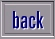Home Page Biology Chemistry Physics Science Superstars Inventions Teachers Stress Homework Help Zephyrus Training About Us Contact Us Credits Disclaimer Privacy Cookies

Question: I need to find out what number goes in the space. Here it is : 2[ ]=13 { note : [ ] means space ]}

Answer: Using the information you sent us, here is a possible solution:

23 = 13

This is because you are changing a base five number to its base ten equivalent.

We don't know how familiar you are with number bases, but we normally count in tens - so it is called a base ten system. The numbers we use are combinations of the numbers 0, 1, 2, 3, 4, 5, 6, 7, 8, 9.
In the base five system, the numbers are 0, 1, 2, 3, 4.
To give you some idea, here are some base ten numbers and their base five equivalents:

 Base 10 Base 5 0 0 1 1 2 2 3 3 4 4 5 10 (this is one zero, not ten) 6 11 (this is one one, not eleven) 7 12 8 13 9 14 10 20 11 21 12 22 13 23 14 24 15 30 etc.

So to change base ten 13 to base five, you have 2 groups of five and 3 units, which gives you

13(base 10) = 23 (base 5)to homework page